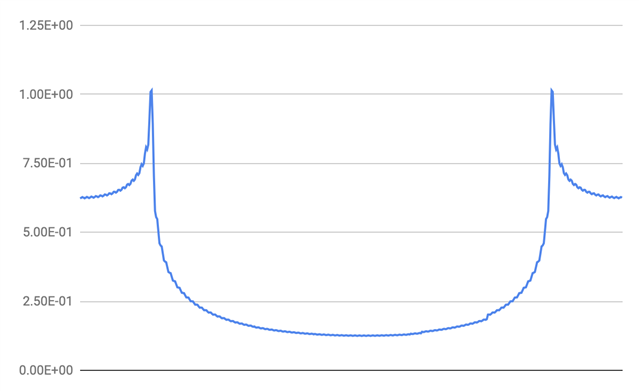# rfft_fast_f32 Not Producing Expected Output

I have been trying to use the RFFT functions in the CMSIS DSP library which has been giving me some confusing outputs.

From the documentation:

Looking at the data, we see that we can uniquely represent the FFT using only N/2 complex numbers. These are packed into the output array in alternating real and imaginary components:

X = { real, imag, real, imag, real, imag ... real[(N/2)-1], imag[(N/2)-1 }

I took this to mean that after running the rfft function on a sequence of N values, the function would output complex values only up to the N/2th value - ie we would only get the first half of the frequency domain output since the other half is just the complex conjugate of the other.

However, what I found was that the function seemingly outputs both halves.

My code is below:

`float32_t H; // FIR filter coefficients`
`float32_t magnitude;`

`arm_rfft_fast_instance_f32 rfftInstance;`
`arm_rfft_fast_init_f32(&rfftInstance, 1024);`

`arm_rfft_fast_f32(&rfftInstance, H, H, 0);`

`arm_cmplx_mag_f32(H, magnitude, 512); // See image below for output`

I am taking a 1024 point FFT on my filter coefficients, H.  Given my assumption above, I would have expected only the first half of the spectrum.

Since the output has interlaced real/imag values, 512 complex values would require a 1024 element array.

When I take the magnitude of the output spectrum (see image), I get both halves which runs counter to my expectation.  Moreover, given that I get both halves within a space of 512 points, it's almost as if I did a 512-point FFT instead of 1024.

I must be missing something here.  Any help is appreciated.Resultant magnitude spectrum.  Array holding this data has 512 elements.  Both halves of the spectrum are present.

More questions in this forum

1665 views
2 replies
Latest
by

1181 views
3 replies
Latest

1144 views
2 replies
Latest